# Convergence to a self-normalized G-Brownian motion

## Abstract

G-Brownian motion has a very rich and interesting new structure that nontrivially generalizes the classical Brownian motion. Its quadratic variation process is also a continuous process with independent and stationary increments. We prove a self-normalized functional central limit theorem for independent and identically distributed random variables under the sub-linear expectation with the limit process being a G-Brownian motion self-normalized by its quadratic variation. To prove the self-normalized central limit theorem, we also establish a new Donsker’s invariance principle with the limit process being a generalized G-Brownian motion.

## Introduction

Let {X n ;n≥1} be a sequence of independent and identically distributed random variables on a probability space. Set $$S_{n}=\sum _{j=1}^{n} X_{j}$$. Suppose EX 1=0 and $$EX_{1}^{2}=\sigma ^{2}>0$$. The well-known central limit theorem says that

$$\frac{S_{n}}{\sqrt{n}}\overset{d}\rightarrow N\left(0,\sigma^{2}\right),$$
(1)

or, equivalently, for any bounded continuous function ψ(x),

$$E\left[\psi\left(\frac{S_{n}}{\sqrt{n}}\right)\right]\rightarrow E\left[\psi(\xi)\right],$$
(2)

where ξN(0,σ 2) is a normal random variable. If the normalization factor $$\sqrt {n}$$ is replaced by $$\sqrt {V_{n}}$$, where $$V_{n}=\sum _{j=1}^{n} X_{j}^{2}$$, then

$$\frac{S_{n}}{\sqrt{V_{n}}}\overset{d}\rightarrow N(0,1).$$
(3)

Giné et al. (1997) proved that (3) holds if and only if EX 1=0 and

$${\lim}_{x\rightarrow \infty} \frac{x^{2}P\left(|X_{1}|\ge x\right)}{EX_{1}^{2}I\{|X_{1}|\le x\}}=0.$$
(4)

The result (3) is refered to as the self-normalized central limit theorem. The purpose of this paper is to establish the self-normalized central limit theorem under the sub-linear expectation.

The sub-linear expectation, or also called G-expectation, is a nonlinear expectation generalizing the notions of backward stochastic differential equations, g-expectations, and provides a flexible framework to model non-additive probability problems and the volatility uncertainty in finance. Peng (2006, 2008a,b) introduced a general framework of the sub-linear expectation of random variables and the notions of the G-normal random variable, G-Brownian motion, independent and identically distributed random variables, etc., under the sub-linear expectation. The construction of sub-linear expectations on the space of continuous paths and discrete-time paths can also be founded in Yan et al. (2012) and Nutz and van Handel (2013). For basic properties of the sub-linear expectation, one can refer to Peng (2008b, 2009, 2010a etc.). For stochastic calculus and stochastic differential equations with respect to a G-Brownian motion, one can refer to Li and Peng (2011), Hu et al. (2014a, b), etc., and a book by Peng (2010a).

The central limit theorem under the sub-linear expectation was first established by Peng (2008b). It says that (2) remains true when the expectation E is replaced by a sub-linear expectation $$\hat {\mathbb {E}}$$ if {X n ;n≥1} are independent and identically distributed under $$\hat {\mathbb {E}}$$, i.e.,

$$\frac{S_{n}}{\sqrt{n}}\overset{d}\rightarrow \xi~\text{under}~\hat{\mathbb{E}},$$
(5)

where ξ is a G-normal random variable.

In the classical case, when $$\textsf {E}[X_{1}^{2}]$$ is finite, (3) follows from the cental limit theorem (1) directly by Slutsky’s lemma and the fact that

$$\frac{V_{n}}{n}\overset{P}\rightarrow \sigma^{2}.$$

The latter is due to the law of large numbers. Under the framework of the sub-linear expectation, $$\frac {V_{n}}{n}$$ no longer converges to a constant. The self-normalized central limit theorem cannot follow from the central limit theorem (5) directly. In this paper, we will prove that

$$\frac{S_{n}}{\sqrt{V_{n}}}\overset{d}\rightarrow \frac{W_{1}}{\sqrt{\langle W\rangle_{1}}}~\text{under}~\hat{\mathbb{E}},$$
(6)

where W t is a G-Brownian motion and 〈W t is its quadratic variation process. A very interesting phenomenon of G-Brownian motion is that its quadratic variation process is also a continuous process with independent and stationary increments, and thus can still be regarded as a Brownian motion. When the sub-linear expectation $$\hat {\mathbb {E}}$$ reduces to a linear one, W t is the classical Brownian motion with W 1N(0,σ 2) and 〈W t =t σ 2, and then (6) is just (3). Our main results on the self-normalized central limit theorem will be given in Section “Main results”, where the process of the self-normalized partial sums $${S_{[nt]}}/{\sqrt {V_{n}}}$$ is proved to converge to a self-normalized G-Brownian motion $${W_{t}}/{\sqrt {\langle W\rangle _{1}}}$$. We also consider the case in which the second moments of X i ’s are infinite and obtain the self-normalized central limit theorem under a condition similar to (4). In the next section, we state basic settings in a sub-linear expectation space, including capacity, independence, identical distribution, G-Brownian motion, etc. One can skip this section if these concepts are familiar. To prove the self-normalized central limit theorem, we establish a new Donsker’s invariance principle in Section “Invariance principle” with the limit process being a generalized G-Brownian motion. The proof is given in the last section.

## Basic settings

We use the framework and notations of Peng (2008b). Let $$(\Omega,\mathcal F)$$ be a given measurable space and letbe a linear space of real functions defined on $$(\Omega,\mathcal F)$$ such that if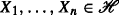, thenfor each $$\varphi \in C_{b}(\mathbb {R}^{n})\bigcup C_{l,Lip}(\mathbb {R}^{n})$$, where $$C_{b}(\mathbb R^{n})$$ denotes the space of all bounded continuous functions and $$C_{l,Lip}(\mathbb {R}^{n})$$ denotes the linear space of (local Lipschitz) functions φ satisfying

$$\begin{array}{@{}rcl@{}} & |\varphi(\boldsymbol{x}) - \varphi(\boldsymbol{y})| \le C(1 + |\boldsymbol{x}|^{m} + |\boldsymbol{y}|^{m})|\boldsymbol{x}- \boldsymbol{y}|, \;\; \forall \boldsymbol{x}, \boldsymbol{y} \in \mathbb R^{n},&\\ & \text {for some}~C > 0, m \in \mathbb N~\text{depending on}~\varphi. & \end{array}$$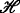is considered as a space of “random variables.” In this case, we denote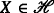. Further, we let $$C_{b,Lip}(\mathbb R^{n})$$ denote the space of all bounded and Lipschitz functions on $$\mathbb R^{n}$$.

### Definition 1

A sub-linear expectation $$\hat {\mathbb {E}}$$ onis a functionsatisfying the following properties: for all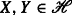, we have

1. (a)

Monotonicity: If XY then $$\hat {\mathbb {E}} [X]\ge \hat {\mathbb {E}} [Y]$$;

2. (b)

Constant preserving:$$\hat {\mathbb {E}} [c] = c$$;

3. (c)

Sub-additivity:$$\hat {\mathbb {E}}[X+Y]\le \hat {\mathbb {E}} [X] +\hat {\mathbb {E}} [Y ]$$ whenever $$\hat {\mathbb {E}} [X] +\hat {\mathbb {E}} [Y ]$$ is not of the form + or −+;

4. (d)

Positive homogeneity:$$\hat {\mathbb {E}} [\lambda X] = \lambda \hat {\mathbb {E}} [X]$$, λ≥0.

Here $$\overline {\mathbb R}=[-\infty, \infty ]$$. The tripleis called a sub-linear expectation space. Given a sub-linear expectation $$\hat {\mathbb {E}}$$, let us denote the conjugate expectation $$\widehat {\mathcal {E}}$$of $$\hat {\mathbb {E}}$$ by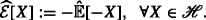Next, we introduce the capacities corresponding to the sub-linear expectations. Let $$\mathcal G\subset \mathcal F$$. A function $$V:\mathcal G\rightarrow [0,1]$$ is called a capacity if

$$V(\emptyset)=0, \;V(\Omega)=1, \;~\text{and}~V(A)\le V(B)\;\; \forall\; A\subset B, \; A,B\in \mathcal G.$$

It is called sub-additive if $$V(A\bigcup B)\le V(A)+V(B)$$ for all $$A,B\in \mathcal G$$ with $$A\bigcup B\in \mathcal G$$.

Letbe a sub-linear space and $$\widehat {\mathcal {E}}$$ be the conjugate expectation of $$\hat {\mathbb {E}}$$. We introduce the pair $$(\mathbb {V},\mathcal {V})$$ of capacities by settingwhere A c is the complement set of A. Then, $$\mathbb {V}$$ is sub-additive and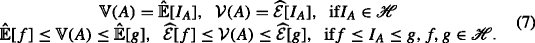Further, we define an extension of $$\hat {\mathbb {E}}^{\ast }$$ of $$\hat {\mathbb {E}}$$ bywhere inf=+. Then,### Definition 2

(Peng (2006, 2008b))

1. (i)

(Identical distribution) Let X 1 and X 2 be two n-dimensional random vectors defined, respectively, in sub-linear expectation spacesand. They are called identically distributed, denoted by $$\boldsymbol X_{1}\overset {d}= \boldsymbol X_{2}$$ if

$$\hat{\mathbb{E}}_{1}[\varphi(\boldsymbol X_{1})]=\hat{\mathbb{E}}_{2}[\varphi(\boldsymbol X_{2})], \;\; \forall \varphi\in C_{l,Lip}(\mathbb R^{n}),$$

whenever the sub-expectations are finite. A sequence {X n ;n≥1} of random variables is said to be identically distributed if $$X_{i}\overset {d}= X_{1}$$ for each i≥1.

2. (ii)

(Independence) In a sub-linear expectation space, a random vectoris said to be independent to another random vector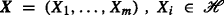under $$\hat {\mathbb {E}}$$ if for each test function $$\varphi \in C_{l,Lip}(\mathbb R^{m} \times \mathbb R^{n})$$ we have

$$\hat{\mathbb{E}} [\varphi(\boldsymbol{X}, \boldsymbol{Y})] = \hat{\mathbb{E}} \left[\hat{\mathbb{E}}[\varphi(\boldsymbol{x}, \boldsymbol{Y})]\big|_{\boldsymbol{x}=\boldsymbol{X}}\right],$$

whenever $$\overline {\varphi }(\boldsymbol {x}):=\hat {\mathbb {E}}\left [|\varphi (\boldsymbol {x}, \boldsymbol {Y})|\right ]<\infty$$ for all x and $$\hat {\mathbb {E}}\left [|\overline {\varphi }(\boldsymbol {X})|\right ]<\infty$$.

3. (iii)

(IID random variables) A sequence of random variables {X n ;n≥1} is said to be independent and identically distributed (IID), if $$X_{i}\overset {d}=X_{1}$$ and X i+1 is independent to (X 1,…,X i ) for each i≥1.

### G-normal distribution, G-Brownian motion and its quadratic variation

Let $$0<\underline {\sigma }\le \overline {\sigma }<\infty$$ and $$G(\alpha)=\frac {1}{2}\left (\overline {\sigma }^{2} \alpha ^{+} - \underline {\sigma }^{2} \alpha ^{-}\right)$$. X is called a normal $$N\left (0, \left [\underline {\sigma }^{2}, \overline {\sigma }^{2}\right ]\right)$$ distributed random variable (written as $$X\sim N\left (0, \left [\underline {\sigma }^{2}, \overline {\sigma }^{2}\right ]\right)$$) under $$\hat {\mathbb {E}}$$, if for any bounded Lipschitz function φ, the function $$u(x,t)=\hat {\mathbb {E}}\left [\varphi \left (x+\sqrt {t} X\right)\right ]$$ ($$x\in \mathbb R, t\ge 0$$) is the unique viscosity solution of the following heat equation:

$$\partial_{t} u -G\left(\partial_{xx}^{2} u\right) =0, \;\; u(0,x)=\varphi(x).$$

Let C[0,1] be a function space of continuous functions on [0,1] equipped with the supremum norm $$\|x\|=\sup \limits _{0\le t\le 1}|x(t)|$$ and C b (C[0,1]) is the set of bounded continuous functions $$h(x):C[0,1]\rightarrow \mathbb R$$. The modulus of the continuity of an element xC[0,1] is defined by

$$\omega_{\delta}(x)=\sup_{|t-s|<\delta}|x(t)-x(s)|.$$

It is showed that there is a sub-linear expectation space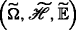with $$\widetilde {\Omega }= C[0,1]$$ and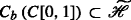such that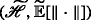is a Banach space, and the canonical process $$W(t)(\omega) = \omega _{t} (\omega \in \widetilde {\Omega })$$ is a G-Brownian motion with $$W(1)\sim N\left (0, \left [\underline {\sigma }^{2}, \overline {\sigma }^{2}\right ]\right)$$ under $$\widetilde {\mathbb E}$$, i.e., for all 0≤t 1<…<t n ≤1, $$\varphi \in C_{l,lip}(\mathbb R^{n})$$,

$$\widetilde{\mathbb E}\left[\varphi\left(W(t_{1}),\ldots, W(t_{n-1}), W(t_{n})-W(t_{n-1})\right)\right] =\widetilde{\mathbb E}\left[\psi\left(W(t_{1}),\ldots, W(t_{n-1})\right)\right],$$
(8)

where $$\psi \left (x_{1},\ldots, x_{n-1}\right)\big)=\widetilde {\mathbb {E}}\left [\varphi \left (x_{1},\ldots, x_{n-1}, \sqrt {t_{n}-t_{n-1}}W(1)\right)\right ]$$ (cf. Peng (2006, 2008a, 2010a), Denis et al. (2011)).

The quadratic variation process of a G-Brownian motion W is defined by

$$\langle W \rangle_{t}={\lim}_{\|\Pi_{t}^{N}\|\rightarrow 0}\sum_{j=1}^{N-1} \left(W\left(t_{j}^{N}\right)-W\left(t_{j-1}^{N}\right)\right)^{2}=W^{2}(t)-2\int_{0}^{t} W(t) dW(t),$$

where $$\Pi _{t}^{N}=\left \{t_{0}^{N},t_{1}^{N},\ldots, t_{N}^{n}\right \}$$ is a partition of [0,t] and $$\left \|\Pi _{t}^{N}\right \|=\max _{j}\left |t_{j}^{N}-t_{j-1}^{N}\right |$$, and the limit is taken in L 2, i.e.,

$${\lim}_{\left\|\Pi_{t}^{N}\right\|\rightarrow 0}\widetilde{\mathbb{E}}\left[\left(\sum_{j=1}^{N-1}\left(W\left(t_{j}^{N}\right)-W\left(t_{j-1}^{N}\right)\right)^{2}-\langle W \rangle_{t}\right)^{2}\right]=0.$$

The quadratic variation process 〈W t is also a continuous process with independent and stationary increments. For the properties and the distribution of the quadratic variation process, one can refer to a book by Peng (2010a).

Denis et al. (2011) showed the following representation of the G-Brownian motion (cf. Theorem 52).

### Lemma 1

Let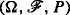be a probability measure space and {B(t)} t≥0 is a P-Brownian motion. Then, for all bounded continuous functions $$\varphi : C_{b}[0,1]\rightarrow \ \mathbb R$$,

$$\widetilde{\mathbb E}\left[\varphi\left(W(\cdot)\right)\right]=\sup_{\theta\in \Theta}\mathsf{E}_{P}\left[\varphi\left(W_{\theta}(\cdot)\right)\right],\;\; W_{\theta}(t) = \int_{0}^{t}\theta(s) dB(s),$$

whereFor the reminder of this paper, the sequences {X n ;n≥1}, {Y n ;n≥1}, etc., of the random variables are considered in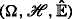. Without specification, we suppose that {X n ;n≥1} is a sequence of independent and identically distributed random variables inwith $$\hat {\mathbb {E}}[X_{1}]=\widehat {\mathcal {E}}[X_{1}]=0$$, $$\hat {\mathbb {E}}\left [X_{1}^{2}\right ]=\overline {\sigma }^{2}$$, and $$\widehat {\mathcal {E}}\left [X_{1}^{2}\right ]=\underline {\sigma }^{2}$$. Denote $$S_{0}^{X}=0$$, $$S_{n}^{X}=\sum _{k=1}^{n} X_{k}$$, V 0=0, $$V_{n}=\sum _{k=1}^{n} X_{k}^{2}$$. And suppose thatis a sub-linear expectation space which is rich enough such that there is a G-Brownian motion W(t) with $$W(1)\sim N\left (0,\left [\underline {\sigma }^{2},\overline {\sigma }^{2}\right ]\right)$$. We denote a pair of capacities corresponding to the sub-linear expectation $$\widetilde {\mathbb E}$$ by $$\left (\widetilde {\mathbb {V}},\widetilde {\mathcal {V}}\right)$$, and the extension of $$\widetilde {\mathbb E}$$ by $$\widetilde {\mathbb {E}}^{\ast }$$.

## Main results

We consider the convergence of the process $$S_{[nt]}^{X}$$. Because it is not in C[0,1], it needs to be modified. Define the C[0,1]-valued random variable $$\widetilde {S}_{n}^{X}(\cdot)$$ by setting

$$\widetilde{S}_{n}^{X}(t)= \left\{\begin{array}{cc} \sum_{j=1}^{k} X_{j}, \; \text{if}~t=k/n \; (k=0,1,\ldots, n);\\ \text{extended by linear interpolation in each interval }\\ \qquad \quad \left[[k-1]n^{-1}, kn^{-1}\right]. \end{array}\right.$$

Then, $$\widetilde {S}_{n}^{X}(t)=S_{[nt]}^{X}+(nt-[nt])X_{[nt]+1}$$. Here [nt] is the largest integer less than or equal to nt. Zhang (2015) obtained the functional central limit theorem as follows.

### Theorem 1

Suppose $$\hat {\mathbb {E}}\left [\left (X_{1}^{2}-b\right)^{+}\right ]\rightarrow 0$$ as b. Then, for all bounded continuous functions $$\varphi :C[0,1]\rightarrow \mathbb {R}$$,

$$\hat{\mathbb{E}}\left[\varphi\left(\frac{\widetilde{S}_{n}^{X}(\cdot)}{\sqrt{n}}\right)\right]\rightarrow \widetilde{\mathbb{E}}\left[{\phantom{2_{1}^{2}}}\!\!\!\!\!\varphi\left(W(\cdot) \right)\! \right].$$
(9)

Replacing the normalization factor $$\sqrt {n}$$ by $$\sqrt {V_{n}}$$, we obtain the self-normalized process of partial sums:

$$W_{n}(t)=\frac{\widetilde{S}_{n}^{X}(t)}{\sqrt{V_{n}}},$$

where $$\frac {0}{0}$$ is defined to be 0. Our main result is the following self-normalized functional central limit theorem (FCLT).

### Theorem 2

Suppose $$\hat {\mathbb {E}}\left [\left (X_{1}^{2}-b\right)^{+}\right ]\rightarrow 0$$ as b. Then, for all bounded continuous functions $$\varphi :C[0,1]\rightarrow \mathbb {R}$$,

$$\hat{\mathbb{E}}^{\ast}\left[\varphi\left(W_{n}(\cdot)\right)\right]\rightarrow\widetilde{\mathbb{E}}\left[\varphi\left(\frac{W(\cdot)}{\sqrt{\langle W \rangle_{1}}}\right) \right].$$
(10)

In particular, for all bounded continuous functions $$\varphi :\mathbb {R}\rightarrow \mathbb {R}$$,

\begin{aligned} \hat{\mathbb{E}}^{\ast}\left[\varphi\left(\frac{S_{n}^{X}}{\sqrt{V_{n}}}\right)\right]\rightarrow & \widetilde{\mathbb{E}}\left[\varphi\left(\frac{W(1)}{\sqrt{\langle W \rangle_{1}}}\right) \right]\\ &=\sup_{\theta\in \Theta}\textsf{E}_{P}\left[\varphi\left(\frac{\int_{0}^{1}\theta(s) d B(s)}{\sqrt{\int_{0}^{1} \theta^{2}(s) ds }}\right) \right]. \end{aligned}
(11)

### Remark 1

It is obvious that

$$\widetilde{\mathbb{E}}\left[\varphi\left(\frac{W(\cdot)}{\sqrt{\langle W \rangle_{1}}}\right) \right] \ge \textsf{E}_{P} \left[\varphi\left(B(\cdot)\right) \right].$$

An interesting problem is how to estimate the upper bounds of the expectations on the right hand side of (10) and (11).

Further, $$\frac {W(\cdot)}{\sqrt {\langle W\rangle _{1}}}\overset {d}=\frac {\overline {W}(\cdot)}{\sqrt {\langle \overline {W}\rangle _{1}}}$$, where $$\overline {W}(t)$$ is a G-Brownian motion with $$\overline {W}(1)\sim N(0,[r^{-2},1])$$, $$r^{2}=\overline {\sigma }^{2}/\underline {\sigma }^{2}$$.

For the classical self-normalized central limit theorem, Giné et al. (1997) showed that the finiteness of the second moments can be relaxed to the condition (4). Csörgő et al. (2003) proved the self-normalized functional central limit theorem under (4). The next theorem gives a similar result under the sub-linear expectation and is an extension of Theorem 2.

### Theorem 3

Let {X n ;n≥1} be a sequence of independent and identically distributed random variables in the sub-linear expectation space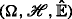with $$\hat {\mathbb {E}}[X_{1}]=\widehat {\mathcal {E}}[X_{1}]=0$$. Denote $$l(x)=\hat {\mathbb {E}}\left [X_{1}^{2}\wedge x^{2}\right ]$$. Suppose

1. (I)

$$x^{2}\mathbb {V}(|X_{1}|\ge x)=o\left (l(x)\right)$$ as x;

2. (II)

$${\lim }_{x\rightarrow \infty } \frac {\hat {\mathbb {E}}\left [X_{1}^{2}\wedge x^{2}\right ]}{\widehat {\mathcal {E}}\left [X_{1}^{2}\wedge x^{2}\right ]}=r^{2}<\infty$$;

3. (III)

$$\hat {\mathbb {E}}[(|X_{1}|-c)^{+}]\rightarrow 0$$ as c.

Then, the conclusions of Theorem 2 remain true with W(t) being a G-Brownian motion such that W(1)N(0,[r −2,1]).

### Remark 2

Note for c>1, $$l(cx)=\hat {\mathbb {E}}\left [X_{1}^{2}\wedge (cx)^{2}\right ]\le l(x)+(cx)^{2}\mathbb {V}(|X_{1}|\ge x)$$. Condition (I) implies that l(cx)/l(x)→1 as x, i.e., l(x) is a slowly varying function. Therefore, there is a constant C such that $$\int _{x}^{\infty }y^{-2}l(y)dy \le C x^{-1} l(x)$$ if x is large enough. So, $$\int _{x}^{\infty }\mathbb {V}(|X_{1}|\ge y)dy=o(x^{-1}l(x))$$. Also, by Lemma 3.9 (b) of Zhang (2016), condition (III) implies that $$\hat {\mathbb {E}}\left [(|X_{1}|-x)^{+}\right ]\le \int _{x}^{\infty }\mathbb {V}(|X_{1}|\ge y)dy$$. Hence, $$\hat {\mathbb {E}}\left [\left (|X_{1}|-x\right)^{+}\right ]=o(x^{-1}l(x))$$ if conditions (I) and (III) are satisfied. When $$\hat {\mathbb {E}}$$ is a continuous sub-linear expectation, then for any random variable Y we have $$\hat {\mathbb {E}}[|Y|]\le \int _{0}^{\infty }\mathbb {V}(|Y|\ge y)dy$$ by Lemma 3.9 (c) of Zhang (2016), and so the condition (III) can be removed. Here, $$\hat {\mathbb {E}}$$ is called continuous if, for any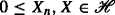with $$\hat {\mathbb {E}}[X_{n}],\hat {\mathbb {E}}[X]<\infty$$, $$\hat {\mathbb {E}}[X_{n}]\nearrow \hat {\mathbb {E}}[X]$$ whenever 0≤X n X, and, $$\hat {\mathbb {E}}[X_{n}]\searrow \hat {\mathbb {E}}[X]$$ whenever X n X.

## Invariance principle

To prove Theorems 2 and 3, we will prove a new Donsker’s invariance principle. Let {(X i ,Y i );i≥1} be a sequence of independent and identically distributed random vectors in the sub-linear expectation spacewith $$\hat {\mathbb {E}}[X_{1}]=\hat {\mathbb {E}}[-X_{1}]=0$$, $$\hat {\mathbb {E}}[X_{1}^{2}]=\overline {\sigma }^{2}$$, $$\widehat {\mathcal {E}}[X_{1}^{2}]=\underline {\sigma }^{2}$$, $$\hat {\mathbb {E}}[Y_{1}]=\overline {\mu }$$, $$\widehat {\mathcal {E}}[Y_{1}]=\underline {\mu }$$. Denote

$$G(p,q)=\hat{\mathbb{E}}\left[\frac{1}{2} q X_{1}^{2}+pY_{1}\right], \;\; p,q\in \mathbb R.$$
(12)

Let ξ be a G-normal distributed random variable, η be a maximal distributed random variable such that the distribution of (ξ,η) is characterized by the following parabolic partial differential equation (PDE) defined on $$[0,\infty)\times \mathbb {R}\times \mathbb {R}$$:

$$\partial_{t} u -G\left(\partial_{y} u, \partial_{xx}^{2} u\right) =0,$$
(13)

i.e., if for any bounded Lipschitz function $$\varphi (x,y):\mathbb {R}^{2}\rightarrow \mathbb {R}$$, the function $$u(x,y,t)=\widetilde {\mathbb {E}}\left [\varphi \left (x+\sqrt {t} \xi, y+t\eta \right)\right ]$$ ($$x,y \in \mathbb {R}, t\ge 0$$) is the unique viscosity solution of the PDE (13) with Cauchy condition u| t=0=φ.

Further, let B t and b t be two random processes such that the distribution of the process (B ·,b ·) is characterized by

1. (i)

B 0=0, b 0=0;

2. (ii)

for any 0≤t 1≤…≤t k st+s, (B s+t B s ,b s+t b s ) is independent to $$(B_{t_{j}}, b_{t_{j}}), j=1,\ldots,k$$, in sense that, for any $$\varphi \in C_{l,Lip}(\mathbb {R}^{2(k+1)})$$,

\begin{aligned} & \widetilde{\mathbb{E}}\left[\varphi\left((B_{t_{1}}, b_{t_{1}}),\ldots,(B_{t_{k}}, b_{t_{k}}), (B_{s+t}-B_{s}, b_{s+t}-b_{s})\right)\right]\\ &\qquad = \widetilde{\mathbb{E}}\left[\psi\left((B_{t_{1}}, b_{t_{1}}),\ldots,(B_{t_{k}}, b_{t_{k}})\right)\right], \end{aligned}
(14)

where

\begin{aligned} \psi\left((x_{1}, y_{1}),\ldots,(x_{k}, y_{k})\right)= \widetilde{\mathbb{E}}\left[\varphi\left((x_{1}, y_{1}),\ldots,(x_{k}, y_{k})\right.\right.,\\ \left.\left.(B_{s+t}-B_{s}, b_{s+t}-b_{s})\right)\right]; \end{aligned}
3. (iii)

for any t,s>0, $$(B_{s+t}-B_{s},b_{s+t}-b_{s})\overset {d}\sim (B_{t},b_{t})$$ under $$\widetilde {\mathbb {E}}$$;

4. (iv)

for any t>0, $$(B_{t},b_{t})\overset {d}\sim \left (\sqrt {t}B_{1}, tb_{1}\right)$$ under $$\widetilde {\mathbb {E}}$$;

5. (v)

the distribution of (B 1,b 1) is characterized by the PDE (13).

It is easily seen that B t is a G-Brownian motion with $$B_{1}\sim N\left (0,[\underline {\sigma }^{2},\overline {\sigma }^{2}]\right)$$, and (B t ,b t ) is a generalized G-Brownian motion introduced by Peng (2010a). The existence of the generalized G-Brownian motion can be found in Peng (2010a).

### Theorem 4

Suppose $$\hat {\mathbb {E}}\left [(X_{1}^{2}-b)^{+}\right ]\rightarrow 0$$ and $$\hat {\mathbb {E}}\left [(|Y_{1}|-b)^{+}\right ]\rightarrow 0$$ as b. Let

$$\widetilde{\boldsymbol{W}}_{n}(t)=\left(\frac{\widetilde{S}_{n}^{X}(t)}{\sqrt{n}}, \frac{\widetilde{S}_{n}^{Y}(t)}{n}\right).$$

Then, for any bounded continuous function $$\varphi :C[0,1]\times C[0,1]\rightarrow \mathbb R$$,

$${\lim}_{n\rightarrow \infty}\hat{\mathbb{E}}\left[\varphi\left(\widetilde{\boldsymbol{W}}_{n}(\cdot) \right)\right]= \widetilde{\mathbb{E}}\left[\varphi\left(B_{\cdot},b_{\cdot}\right)\right].$$
(15)

Further, let p≥2, q≥1, and assume $$\hat {\mathbb {E}}[|X_{1}|^{p}]<\infty$$, $$\hat {\mathbb {E}}[|Y_{1}|^{q}]<\infty$$. Then, for any continuous function $$\varphi :C[0,1]\times C[0,1]\rightarrow \mathbb R$$ with |φ(x,y)|≤C(1+xp+yq),

$${\lim}_{n\rightarrow \infty}\hat{\mathbb{E}}^{\ast}\left[\varphi\left(\widetilde{\boldsymbol{W}}_{n}(\cdot) \right)\right]= \widetilde{\mathbb{E}}\left[\varphi\left(B_{\cdot},b_{\cdot}\right)\right].$$
(16)

Here x= sup0≤t≤1|x(t)| for xC[0,1].

### Remark 3

When X k and Y k are random vectors in $$\mathbb R^{d}$$ with $$\hat {\mathbb {E}}[X_{k}]=\hat {\mathbb {E}}[-X_{k}]=0$$, $$\hat {\mathbb {E}}[(\|X_{1}\|^{2}-b)^{+}]\rightarrow 0$$ and $$\hat {\mathbb {E}}[(\|Y_{1}\|-b)^{+}]\rightarrow 0$$ as b. Then, the function G in (12) becomes

$$G(p,A)=\hat{\mathbb{E}}\left[\frac{1}{2}\langle AX_{1},X_{1}\rangle+\langle p,Y_{1}\rangle\right],\;\; p\in \mathbb R^{d}, A\in\mathbb S(d),$$

where $$\mathbb S(d)$$ is the collection of all d×d symmetric matrices. The conclusion of Theorem 4 remains true with the distribution of (B 1,b 1) being characterized by the following parabolic partial differential equation defined on $$[0,\infty)\times \mathbb {R}^{d}\times \mathbb {R}^{d}$$:

$$\partial_{t} u -G\left(D_{y} u, D_{xx}^{2} u\right) =0,\;\; u|_{t=0}=\varphi,$$

where $$D_{y} =(\partial _{y_{i}})_{i=1}^{n}$$ and $$D_{xx}^{2}=(\partial _{x_{i}x_{j}}^{2})_{i,j=1}^{d}$$.

### Remark 4

As a conclusion of Theorem 4, we have

$$\hat{\mathbb{E}}\left[\varphi\left(\frac{S_{n}^{X}}{\sqrt{n}},\frac{S_{n}^{Y}}{n}\right)\right]\rightarrow \widetilde{\mathbb{E}}\left[{\phantom{2_{0}^{0}}}\!\!\!\!\!\varphi(B_{1},b_{1})\right],\;\; \varphi\in C_{b}(\mathbb{R}^{2}).$$

This is proved by Peng (2010a) under the conditions $$\hat {\mathbb {E}}\left [\left |X_{1}\right |^{2+\delta }\right ]<\infty$$ and $$\hat {\mathbb {E}}\left [|Y_{1}|^{1+\delta }\right ]<\infty$$ (cf. Theorem 3.6 and Remark 3.8 therein).

When Y 1≡0, (15) becomes

$${\lim}_{n\rightarrow \infty}\hat{\mathbb{E}}\left[\varphi\left(\frac{\widetilde{S}_{n}^{X}(\cdot)}{\sqrt{n}}\right)\right]=\widetilde{\mathbb{E}} \left[\varphi\left(B_{\cdot}\right)\right],\;\; \varphi\in C_{b}(C[0,1]),$$

which is proved by Zhang (2015).

Before the proof, we need several lemmas. For random vectors X n in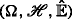and X in, we write $$\boldsymbol X_{n}\overset {d}\rightarrow \boldsymbol {X}$$ if

$$\hat{\mathbb{E}}\left[\varphi(\boldsymbol{X}_{n})\right]\rightarrow \widetilde{\mathbb{E}}\left[\varphi(\boldsymbol{X})\right]$$

for any bounded continuous φ. Write $$\boldsymbol X_{n} \overset {\mathbb {V}}\rightarrow \boldsymbol {x}$$ if $$\mathbb {V}(\|\boldsymbol {X}_{n}-\boldsymbol {x}\|\ge \epsilon)\rightarrow 0$$ for any ε>0. {X n } is called uniformly integrable if

$${\lim}_{b\rightarrow \infty}\limsup_{n\rightarrow \infty} \hat{\mathbb{E}}\left[(\|\boldsymbol{X}_{n}\|-b)^{+}\right]= 0.$$

The following three lemmas are obvious.

### Lemma 2

If $$\boldsymbol {X}_{n}\overset {d}\rightarrow \boldsymbol {X}$$ and φ is a continuous function, then $$\varphi (\boldsymbol {X}_{n})\overset {d}\rightarrow \varphi (\boldsymbol {X})$$.

### Lemma 3

(Slutsky’s Lemma) Suppose $$\boldsymbol {X}_{n}\overset {d}\rightarrow \boldsymbol {X}$$, $$\boldsymbol {Y}_{n} \overset {\mathbb {V}}\rightarrow \boldsymbol {y}$$, $$\eta _{n}\overset {\mathbb {V}}\rightarrow a$$, where a is a constant and y is a constant vector, and $$\widetilde {\mathbb {V}}(\|\boldsymbol {X}\|>\lambda)\rightarrow 0$$ as λ. Then, $$(\boldsymbol {X}_{n}, \boldsymbol {Y}_{n}, \eta _{n})\overset {d}\rightarrow (\boldsymbol {X},\boldsymbol {y}, a)$$, and as a result, $$\eta _{n}\boldsymbol {X}_{n}+\boldsymbol {Y}_{n}\overset {d}\rightarrow a\boldsymbol {X}+\boldsymbol {y}$$.

### Remark 5

Suppose $$\boldsymbol {X}_{n}\overset {d}\rightarrow \boldsymbol {X}$$. Then, $$\widetilde {\mathbb {V}}(\|\boldsymbol {X}\|>\lambda)\rightarrow 0$$ as λ is equivalent to the tightness of {X n ;n≥1}, i.e.,

$${\lim}_{\lambda\rightarrow \infty} \limsup_{n\rightarrow \infty} \mathbb{V}\left(\|\boldsymbol{X}_{n}\|>\lambda\right)=0,$$

because for all ε>0, we can define a continuous function φ(x) such that I{x>λ+ε}≤φ(x)≤I{x>λ] and so

\begin{aligned} &\widetilde{\mathbb{V}}(\|\boldsymbol{X}\|>\lambda+\epsilon)\le \widetilde{\mathbb{E}}[\varphi(\|\boldsymbol{X}\|)]={\lim}_{n\rightarrow \infty} \hat{\mathbb{E}}[\varphi(\|\boldsymbol{X}_{n}\|)] \le \limsup_{n\rightarrow \infty} \mathbb{V}\left(\|\boldsymbol{X}_{n}\|> \lambda\right), \\ &\limsup_{n\rightarrow \infty} \mathbb{V}\left(\|\boldsymbol{X}_{n}\|> \lambda+\epsilon\right)\le {\lim}_{n\rightarrow \infty} \hat{\mathbb{E}}[\varphi(\|\boldsymbol{X}_{n}\|)]=\widetilde{\mathbb{E}}[\varphi(\|\boldsymbol{X}\|)] \le \widetilde{\mathbb{V}}(\|\boldsymbol{X}\|> \lambda). \end{aligned}

### Lemma 4

Suppose $$\boldsymbol {X}_{n}\overset {d}\rightarrow \boldsymbol {X}$$.

1. (a)

If {X n } is uniformly integrable and $$\widetilde {\mathbb {E}}[((\|\boldsymbol {X}\|-b)^{+}]\rightarrow 0$$ as b, then,

$$\hat{\mathbb{E}}[\boldsymbol{X}_{n}]\rightarrow \widetilde{\mathbb{E}}[\boldsymbol{X}].$$
(17)
2. (b)

If $$\sup _{n}\hat {\mathbb {E}}[|\boldsymbol X_{n}\|^{q}<\infty$$ and $$\widetilde {\mathbb {E}} [|\boldsymbol {X}\|^{q}<\infty$$ for some q>1, then (17) holds.

The following lemma is proved by Zhang (2015).

### Lemma 5

Suppose that $$\boldsymbol {X}_{n}\overset {d}\rightarrow \boldsymbol {X}$$, $$\boldsymbol {Y}_{n}\overset {d}\rightarrow \boldsymbol {Y}$$, Y n is independent to X n under $$\hat {\mathbb {E}}$$ and $$\widetilde {\mathbb {V}}(\|\boldsymbol {X}\|>\lambda)\rightarrow 0$$ and $$\widetilde {\mathbb {V}}(\|\boldsymbol {Y}\|>\lambda)\rightarrow 0$$ as λ. Then $$(\boldsymbol {X}_{n},\boldsymbol {Y}_{n})\overset {d}\rightarrow (\overline {\boldsymbol {X}},\overline {\boldsymbol {Y}}),$$ where $$\overline {\boldsymbol {X}}\overset {d}=\boldsymbol {X}$$, $$\overline {\boldsymbol {Y}}\overset {d}=\boldsymbol {Y}$$ and $$\overline {\boldsymbol {Y}}$$ is independent to $$\overline {\boldsymbol {X}}$$ under $$\widetilde {\mathbb {E}}$$.

The next lemma is about the Rosenthal-type inequalities due to Zhang (2016).

### Lemma 6

Let {X 1,…,X n } be a sequence of independent random variables in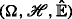.

1. (a)

Suppose p≥2. Then,

\begin{aligned} \hat{\mathbb{E}}\left[\max_{k\le n} \left|S_{k}\right|^{p}\right]&\le C_{p}\left\{ \sum_{k=1}^{n} \hat{\mathbb{E}} \left[|X_{k}|^{p}\right]+\left(\sum_{k=1}^{n} \hat{\mathbb{E}} \left[|X_{k}|^{2}\right]\right)^{p/2} \right. \\ & \qquad \left. +\left(\sum_{k=1}^{n} \left[\left(\widehat{\mathcal{E}} [X_{k}]\right)^{-}+\left(\hat{\mathbb{E}} [X_{k}]\right)^{+}\right]\right)^{p}\right\}. \end{aligned}
(18)
2. (b)

Suppose $$\hat {\mathbb {E}}[X_{k}]\le 0$$, k=1,…,n. Then,

$$\hat{\mathbb{E}}\left[\left|\max_{k\le n} (S_{n}-S_{k})\right|^{p}\right] \le 2^{2-p}\sum_{k=1}^{n} \hat{\mathbb{E}} [|X_{k}|^{p}], \;\; \text{for} 1\le p\le 2$$
(19)

and

\begin{aligned} \hat{\mathbb{E}}\left[\left|\max_{k\le n}(S_{n}- S_{k})\right|^{p}\right] &\le C_{p}\left\{ \sum_{k=1}^{n} \hat{\mathbb{E}} \left[|X_{k}|^{p}\right]+\left(\sum_{k=1}^{n} \hat{\mathbb{E}} \left[|X_{k}|^{2}\right]\right)^{p/2}\right\} \\ &\le C_{p} n^{p/2-1} \sum_{k=1}^{n} \hat{\mathbb{E}} [|X_{k}|^{p}], \;\; \text{for}~p\ge 2. \end{aligned}
(20)

### Lemma 7

Suppose $$\hat {\mathbb {E}}[X_{1}]=\hat {\mathbb {E}}[-X_{1}]=0$$ and $$\hat {\mathbb {E}}\left [X_{1}^{2}\right ]<\infty$$. Let $$\overline {X}_{n,k}=(-\sqrt {n})\vee X_{k}\wedge \sqrt {n}$$, $$\widehat {X}_{n,k}=X_{k}-\overline {X}_{n,k}$$, $$\overline {S}_{n,k}^{X}=\sum _{j=1}^{k} \overline {X}_{n,j}$$ and $$\widehat {S}_{n,k}^{X}=\sum _{j=1}^{k}\widehat {X}_{n,j}$$, k=1,…,n. Then

\begin{aligned} \hat{\mathbb{E}}\left[\max_{k\le n} \left|\frac{\overline{S}_{n,k}^{X}}{\sqrt{n}}\right|^{q}\right]\le C_{q}, \;\; ~\text{for all} ~q\ge 2, \end{aligned}

and

$${\lim}_{n\rightarrow \infty} \hat{\mathbb{E}}\left[\max_{k\le n} \left|\frac{\widehat{S}_{n,k}^{X}}{\sqrt{n}}\right|^{p}\right]=0$$

whenever $$\hat {\mathbb {E}}[(|X_{1}|^{p}-b)^{+}]\rightarrow 0$$ as b if p=2, and $$\hat {\mathbb {E}}[|X_{1}|^{p}]<\infty$$ if p>2.

### Proof

Note $$\hat {\mathbb {E}}[X_{1}]=\widehat {\mathcal {E}}[X_{1}]=0$$. So, $$|\widehat {\mathcal {E}}[\overline {X}_{n,1}]|=|\widehat {\mathcal {E}}[X_{1}]-\widehat {\mathcal {E}}[\overline {X}_{n,1}]|\le \hat {\mathbb {E}}|\widehat {X}_{n,1}|\le \hat {\mathbb {E}}[(|X_{1}|^{2}-n)^{+}]n^{-1/2}$$ and $$|\hat {\mathbb {E}}[\overline {X}_{n,1}]|=|\hat {\mathbb {E}}[X_{1}]-\hat {\mathbb {E}}[\overline {X}_{n,1}]|\le \hat {\mathbb {E}}|\widehat {X}_{n,1}|\le \hat {\mathbb {E}}[(|X_{1}|^{2}-n)^{+}]n^{-1/2}$$. By Rosenthal’s inequality (cf. (18)),

\begin{aligned} & \hat{\mathbb{E}}\left[\max_{k\le n} \left|\overline{S}_{n,k}^{X}\right|^{q}\right] \le C_{p}\left\{ n \hat{\mathbb{E}} [|\overline{X}_{n,1}|^{q}+\left(n \hat{\mathbb{E}} \left[|\overline{X}_{n,1}|^{2}\right]\right)^{q/2}\right.\\ & \qquad\qquad \qquad\qquad \left.+\left(n\left[\left(\widehat{\mathcal{E}} [\overline{X}_{n,1}]\right)^{-}+\left(\hat{\mathbb{E}} [\overline{X}_{n,1}]\right)^{+}\right]\right)^{q}\right\}\\ & \;\; \le C_{q}\left\{ n n^{q/2-1}\hat{\mathbb{E}} \left[|X_{1}|^{2}\right]+n^{q/2}\left(\hat{\mathbb{E}} \left[X_{1}^{2}\right]\right)^{q/2}+\left(nn^{-1/2}\hat{\mathbb{E}}\left[\left(X_{1}^{2}-n\right)^{+}\right]\right)^{q}\right\} \\ & \;\; \le C_{q} n^{q/2}\left\{\hat{\mathbb{E}} \left[\left|X_{1}\right|^{2}\right]+\left(\hat{\mathbb{E}} \left[X_{1}^{2}\right]\right)^{q}\right\}, \;\; \text{for all~} q\ge 2 \end{aligned}

and

\begin{aligned} \hat{\mathbb{E}}\left[\max_{k\le n} \left|\widehat{S}_{n,k}^{X}\right|^{p}\right] \le & C_{p}\left\{ n \hat{\mathbb{E}} \left[|\widehat{X}_{n,1}|^{p}\right]+\left(n \hat{\mathbb{E}} \left[|\widehat{X}_{n,1}|^{2}\right]\right)^{p/2}\right. \\ & \left.\qquad +\left(n\left[\left(\widehat{\mathcal{E}} [\widehat{X}_{n,1}]\right)^{-}+\left(\hat{\mathbb{E}} [\widehat{X}_{n,1}]\right)^{+}\right]\right)^{p}\right\}\\ \le & C_{p}\left\{ n\hat{\mathbb{E}} \left[\big(|X_{1}|^{p}-n^{p/2}\big)^{+}\right]+n^{p/2}\left(\hat{\mathbb{E}} \left[(X_{1}^{2}-n)^{+}\right]\right)^{p/2}\right.\\ & \left.\qquad +n^{p/2}\left(\hat{\mathbb{E}} \left[(X_{1}^{2}-n)^{+}\right]\right)^{p}\right\},\; p\ge 2. \end{aligned}

The proof is completed. □

### Lemma 8

(a) Suppose p≥2, $$\hat {\mathbb {E}}[X_{1}]=\hat {\mathbb {E}}[-X_{1}]=0$$, $$\hat {\mathbb {E}}[(X_{1}^{2}-b)^{+}]\rightarrow 0$$ as b and $$\hat {\mathbb {E}}[|X_{1}|^{p}]<\infty$$. Then,

$$\left\{\max_{k\le n}\left|\frac{S_{k}^{X}}{\sqrt{n}}\right|^{p}\right\}_{n=1}^{\infty} \; \text{is uniformly integrable and therefore is tight}.$$

(b) Suppose p≥1, $$\hat {\mathbb {E}}\left [(|Y_{1}|-b)^{+}\right ]\rightarrow 0$$ as b, and $$\hat {\mathbb {E}}[|Y_{1}|^{p}]<\infty$$. Then,

$$\left\{\max_{k\le n}\left|\frac{S_{k}^{Y}}{n}\right|^{p}\right\}_{n=1}^{\infty} \; \text{is uniformly integrable and therefore is tight}.$$

### Proof

(a) follows from Lemma 6. (b) is obvious by noting

\begin{aligned} & \hat{\mathbb{E}}\left[\left(\left(\frac{\max_{k\le n}|S_{k}^{Y}|}{n}-b\right)^{+}\right)^{p}\right] \le \hat{\mathbb{E}}\left[\left(\frac{\sum_{k=1}^{n} (|Y_{k}|-b)^{+}}{n}\right)^{p}\right]\\ \le& C_{p} \left(\frac{\sum_{k=1}^{n} \hat{\mathbb{E}}[(|Y_{k}|-b)^{+}]}{n}\right)^{p} \\ & \qquad +C_{p} \frac{\hat{\mathbb{E}}\Big[\Big|\left(\sum_{k=1}^{n}\{ (|Y_{k}|-b)^{+}-\hat{\mathbb{E}}[(|Y_{k}|-b)^{+}]\}\right)^{+}\Big|^{p}\Big]}{n^{p}}\\ \le & C_{p}\left(\hat{\mathbb{E}}\left[(|Y_{1}|-b)^{+}\right]\right)^{p}+C_{p}\big(n^{-p/2}+n^{1-p}\big)\hat{\mathbb{E}}\big[(|Y_{1}|^{p}-b^{p})^{+}\big] \end{aligned}

by the Rosenthal-type inequalities (19) and (20). □

### Lemma 9

Suppose $$\hat {\mathbb {E}}\left [(|Y_{1}|-b)^{+}\right ]\rightarrow 0$$ as b. Then, for any ε>0,

$$\mathbb{V}\left(\frac{S_{n}^{Y}}{n}>\hat{\mathbb{E}}[Y_{1}]+\epsilon\right)\rightarrow 0~\text{and}~\mathbb{V}\left(\frac{S_{n}^{Y}}{n}<\widehat{\mathcal{E}}[Y_{1}]-\epsilon\right)\rightarrow 0.$$

### Proof

Let Y k,b =(−b)Y k b, $$S_{n,1}=\sum _{k=1}^{n} Y_{k,b}$$ and $$S_{n,2}=S_{n}^{Y}-S_{n,1}$$. Note $$\hat {\mathbb {E}}\big [Y_{1,b}]\rightarrow \hat {\mathbb {E}}[Y_{1}]$$ as b. Suppose $$\left |\hat {\mathbb {E}}[Y_{1,b}] - \hat {\mathbb {E}}[Y_{1}]\right |<\epsilon /4$$. Then, by Kolmogorov’s inequality (cf. (19)),

\begin{aligned} &\mathbb{V}\left(\frac{S_{n,1}}{n}>\hat{\mathbb{E}}[Y_{1}]+\epsilon/2\right)\le \mathbb{V}\left(\frac{S_{n,1}}{n}>\hat{\mathbb{E}}[Y_{1,b}]+\epsilon/4\right)\\ \le & \frac{16}{n^{2}\epsilon^{2}} \hat{\mathbb{E}}\left[\left(\Big(\sum_{k=1}^{n} \big(Y_{k,b}-\hat{\mathbb{E}}[Y_{k,b}]\big)\Big)^{+}\right)^{2}\right] \\ \le & \frac{32}{n^{2}\epsilon^{2}} \sum_{k=1}^{n} \hat{\mathbb{E}}\left[\big(Y_{k,b}-\hat{\mathbb{E}}[Y_{k,b}]\big)^{2}\right]\le \frac{32(2b)^{2}}{n\epsilon^{2}}\rightarrow 0. \end{aligned}

Also,

\begin{aligned} \mathbb{V}\left(\frac{S_{n,2}}{n}> \epsilon/2\right)\le & \frac{2}{n\epsilon} \sum_{k=1}^{n} \hat{\mathbb{E}}|Y_{k}-Y_{k,b}| \le \frac{2}{\epsilon}\hat{\mathbb{E}}\left[(|Y_{1}|-b)^{+}\right] \rightarrow 0~\text{as}~b \rightarrow \infty. \end{aligned}

It follows that

$$\mathbb{V}\left(\frac{S_{n}^{Y}}{n}>\hat{\mathbb{E}}[Y_{1}]+\epsilon\right)\rightarrow 0.$$

By considering {−Y k } instead, we have

$$\mathbb{V}\left(\frac{S_{n}^{Y}}{n}<\widehat{\mathcal{E}}[Y_{1}]-\epsilon\right)=\mathbb{V}\left(\frac{-S_{n}^{Y}}{n}>\hat{\mathbb{E}}[-Y_{1}]+\epsilon\right)\rightarrow 0.$$

### Proof of Theorem 4.

We first show the tightness of $$\widetilde {\boldsymbol W}_{n}$$. It is easily seen that

$$w_{\delta}\left(\frac{\widetilde{S}_{n}^{Y}(\cdot)}{n}\right) \le 2\delta b+\frac{\sum_{k=1}^{n} (|Y_{k}|-b)^{+}}{n}.$$

It follows that for any ε>0, if δ<ε/(4b), then

$$\sup_{n}\mathbb{V}\left(w_{\delta}\left(\frac{\widetilde{S}_{n}^{Y}(\cdot)}{n}\right)\ge \epsilon\right) \le \sup_{n} \mathbb{V}\left(\sum_{k=1}^{n} (|Y_{k}|-b)^{+}\ge n\frac{\epsilon}{2}\right)\le \frac{2}{\epsilon}\hat{\mathbb{E}}\left[(|Y_{1}|-b)^{+}\right].$$

Letting δ→0 and then b yields

$$\sup_{n}\mathbb{V}\left(w_{\delta}\left(\frac{\widetilde{S}_{n}^{Y}(\cdot)}{n}\right)\ge \epsilon\right)\rightarrow 0~\text{as}~\delta \rightarrow 0.$$

For any η>0, we choose δ k 0 such that, if

$$A_{k}=\left\{x: \omega_{\delta_{k}}(x)<\frac{1}{k}\right\},$$

then $$\sup _{n}\mathbb {V}\left (\widetilde {S}_{n}^{Y}(\cdot)/n \in A_{k}^{c}\right)\le \eta /2^{k+1}$$. Let A={x:|x(0)|≤a}, $$K_{2}=A\bigcap _{k=1}^{\infty }A_{k}$$. Then, by the Arzelá-Ascoli theorem, K 2C b (C[0,1]) is compact. It is obvious that $$\{ \widetilde {S}_{n}^{Y}(\cdot)/n\not \in A\}=\emptyset$$, because $$\widetilde {S}_{n}^{Y}(0)/n=0$$. Next, we show that

$$\mathbb{V}\left(\widetilde{S}_{n}^{Y}(\cdot)/n\in K_{2}^{c}\right)\le \mathbb{V}\left(\widetilde{S}_{n}^{Y}(\cdot)/n\in A^{c}\right)+\sum_{k=1}^{\infty}\mathbb{V}\left(\widetilde{S}_{n}^{Y}(\cdot)/n\in A_{k}^{c}\right).$$

Note that when δ<1/(2n),

$$\omega_{\delta}\left(\widetilde{S}_{n}^{Y}(\cdot)/n\right)\le 2n |t-s|\max_{i\le n} |Y_{i}|/n \le 2 \delta \max_{i\le n} |Y_{i}|.$$

Choose a k 0 such that δ k <1/(2Mk) for kk 0. Then, on the event E={maxin|Y i |≤M}, $$\{ \widetilde {S}_{n}^{Y}(\cdot)/n\in A_{k}^{c}\}=\emptyset$$ for kk 0. So, by the (finite) sub-additivity of $$\mathbb {V}$$,

\begin{aligned} &\mathbb{V}\left(E \bigcap \left\{ \widetilde{S}_{n}^{Y}(\cdot)/n\in K^{c}\right\}\right)\\ \le & \mathbb{V}\left(E \bigcap\left\{ \widetilde{S}_{n}^{Y}(\cdot)/n \in A^{c}\right\}\right)+\sum_{k=1}^{k_{0}}\mathbb{V}\left(E\bigcap \left\{ \widetilde{S}_{n}^{Y}(\cdot)/n \in A_{k}^{c}\right\}\right) \\ \le & \mathbb{V}\left(\widetilde{S}_{n}^{Y}(\cdot)/n \in A^{c}\right)+\sum_{k=1}^{\infty}\mathbb{V} \left(\widetilde{S}_{n}^{Y}(\cdot)/n \in A_{k}^{c}\right). \end{aligned}

On the other hand,

$$\mathbb{V}(E^{c})\le \frac{\hat{\mathbb{E}}[\max_{i\le n} |Y_{i}|]}{M}\le \frac{n\hat{\mathbb{E}}[|Y_{1}|]}{M}.$$

It follows that

\begin{aligned} \mathbb{V}\left(\widetilde{S}_{n}^{Y}(\cdot)/n\in K_{2}^{c} \right) \le \mathbb{V}\left(\widetilde{S}_{n}^{Y}(\cdot)/n \in A^{c}\right)+\sum_{k=1}^{\infty}\mathbb{V}\left(\widetilde{S}_{n}^{Y}(\cdot)/n \in A_{k}^{c}\right)+ \frac{n \hat{\mathbb{E}}[|Y_{1}|]}{M}. \end{aligned}

Letting M yields

\begin{aligned} \mathbb{V}\left(\widetilde{S}_{n}^{Y}(\cdot)/n\in K_{2}^{c} \right) & \le \mathbb{V}(\widetilde{S}_{n}^{Y}(\cdot)/n \in A^{c})+\sum_{k=1}^{\infty}\mathbb{V}\left(\widetilde{S}_{n}^{Y}(\cdot)/n \in A_{k}^{c}\right)\\ &< 0+\sum_{k=1}^{\infty} \frac{\eta}{2^{k+1}}<\frac{\eta}{2}. \end{aligned}

We conclude that for any η>0, there exists a compact K 2C b (C[0,1]) such that

$$\sup_{n} \hat{\mathbb{E}}^{\ast}\left[I\left\{\frac{\widetilde{S}_{n}^{Y}(\cdot)}{n}\not\in K_{2}\right\}\right]=\sup_{n} \mathbb{V}\left \{\frac{\widetilde{S}_{n}^{Y}(\cdot)}{n}\not\in K_{2}\right\}<\eta/2.$$
(21)

Next, we show that for any η>0, there exists a compact K 1C b (C[0,1]) such that

$$\sup_{n} \hat{\mathbb{E}}^{\ast}\left[I\left\{\frac{\widetilde{S}_{n}^{X}(\cdot)}{\sqrt{n}}\not\in K_{1}\right\}\right]=\sup_{n} \mathbb{V}\left \{\frac{\widetilde{S}_{n}^{X}(\cdot)}{\sqrt{n}}\not\in K_{1}\right\}<\eta/2.$$
(22)

Similar to (21), it is sufficient to show that

$$\sup_{n}\mathbb{V}\left(w_{\delta}\left(\frac{\widetilde{S}_{n}^{X}(\cdot)}{\sqrt{n}}\right)\ge \epsilon\right)\rightarrow 0 ~\text{as}~\delta \rightarrow 0.$$
(23)

With the same argument of Billingsley (1968, Pages 56–59, cf. (8.12)), for large n,

\begin{aligned} &\mathbb{V}\left(w_{\delta}\left(\frac{\widetilde{S}_{n}^{X}(\cdot)}{\sqrt{n}}\right)\ge 3\epsilon\right) \le \frac{2}{\delta} \mathbb{V}\left(\max_{i\le [n\delta]} \frac{|S_{i}^{X}|}{\sqrt{[n\delta]}}\ge \epsilon \frac{\sqrt{n}}{\sqrt{[n\delta]}} \right) \\ \le &\frac{2}{\delta} \mathbb{V}\left(\max_{i\le [n\delta]} \frac{\left|S_{i}^{X}\right|}{\sqrt{[n\delta]}}\ge \frac{\epsilon }{\sqrt{2 \delta }} \right) \le \frac{4}{\epsilon^{2}}\hat{\mathbb{E}}\left[\left(\max_{i\le [n\delta]} \Big|\frac{S_{i}^{X}}{\sqrt{[n\delta]}}\Big|^{2}-\frac{\epsilon^{2} }{ 2 \delta }\right)^{+}\right]. \end{aligned}

It follows that

$${\lim}_{\delta\rightarrow 0} \limsup_{n\rightarrow \infty} \mathbb{V}\left(w_{\delta}\left(\frac{\widetilde{S}_{n}^{X}(\cdot)}{\sqrt{n}}\right)\ge 3\epsilon\right)=0$$

by Lemma 8 (a), where p=2. On the other hand, for fixed n, if δ<1/(2n), then

$$\omega_{\delta}(\widetilde{S}_{n}^{X}(\cdot)/\sqrt{n})\le 2n |t-s|\max_{i\le n} |X_{i}|/\sqrt{n} \le 2 \delta \sqrt{n} \max_{i\le n} |X_{i}|.$$

We have

$${\lim}_{\delta\rightarrow 0} \mathbb{V}\left(w_{\delta}\left(\frac{\widetilde{S}_{n}^{X}(\cdot)}{\sqrt{n}}\right)\ge \epsilon\right)=0$$

for each n. It follows that (23) holds.

Now, by combining (21) and (22) we obtain the tightness of $$\widetilde {\boldsymbol W}_{n}$$ as follows.

$$\sup_{n} \hat{\mathbb{E}}^{\ast}\Big[I\Big\{\widetilde{\boldsymbol W}_{n}(\cdot)\not\in K_{1}\times K_{2}\Big\}\Big]<\eta.$$
(24)

Define $$\hat {\mathbb {E}}_{n}$$ by

$$\hat{\mathbb{E}}_{n}[\varphi]=\hat{\mathbb{E}}\Big[\varphi\big(\widetilde{\boldsymbol W}_{n}(\cdot)\big)\Big],\;\; \varphi\in C_{b}\big(C[0,1]\times C[0,1]\big).$$

Then, the sequence of sub-linear expectations $$\{\hat {\mathbb {E}}_{n}\}_{n=1}^{\infty }$$ is tight by (24). By Theorem 9 of Peng (2010b), $$\{\hat {\mathbb {E}}_{n}\}_{n=1}^{\infty }$$ is weakly compact, namely, for each subsequence $$\{\hat {\mathbb {E}}_{n_{k}}\}_{k=1}^{\infty }$$, n k , there exists a further subsequence $$\left \{\hat {\mathbb {E}}_{m_{j}}\right \}_{j=1}^{\infty } \subset \left \{\hat {\mathbb {E}}_{n_{k}}\right \}_{k=1}^{\infty }$$, m j , such that, for each φC b (C[0,1]×C[0,1]), $$\{\hat {\mathbb {E}}_{m_{j}}[\varphi ]\}$$ is a Cauchy sequence. Define $${\mathbb F}[\cdot ]$$ by

$${\mathbb F}[\varphi]={\lim}_{j\rightarrow \infty}\hat{\mathbb{E}}_{m_{j}}[\varphi], \; \varphi\in C_{b}\big(C[0,1]\times C[0,1]\big).$$

Let $$\overline {\Omega }=C[0,1]\times C[0,1]$$, and (ξ t ,η t ) be the canonical process $$\xi _{t}(\omega) = \omega _{t}^{(1)}$$, $$\eta _{t}(\omega)=\omega _{t}^{(2)}\left (\omega =\left (\omega ^{(1)},\omega ^{(2)}\right)\in \overline {\Omega }\right)$$. Then,

$$\hat{\mathbb{E}}\Big[\varphi\big(\widetilde{\boldsymbol W}_{m_{j}}(\cdot)\big)\Big]\rightarrow {\mathbb F}\left[\varphi(\xi_{\cdot},\eta_{\cdot})\right],\;\;\varphi\in C_{b}\big(C[0,1]\times C[0,1]\big).$$
(25)

The topological completion of $$C_{b}(\overline {\Omega })$$ under the Banach norm $${\mathbb F}[\|\cdot \|]$$ is denoted by $$L_{\mathbb F} (\overline {\Omega })$$. $${\mathbb F}[\cdot ]$$ can be extended uniquely to a sub-linear expectation on $$L_{\mathbb F} (\overline {\Omega })$$.

Next, it is sufficient to show that (ξ t ,η t ) defined on the sub-linear space $$(\overline {\Omega }, L_{\mathbb F} (\overline {\Omega }), {\mathbb F})$$ satisfies (i)-(v) and so $$(\xi _{\cdot },\eta _{\cdot })\overset {d}=(B_{\cdot },b_{\cdot })$$, which means that the limit distribution of any subsequence of $$\widetilde {\boldsymbol W}_{n}(\cdot)$$ is uniquely determined.

The conclusion in (i) is obvious. For (ii) and (iii), we let 0≤t 1≤…≤t k st+s. By (25), for any bounded continuous function $$\varphi :\mathbb R^{2(k+1)}\rightarrow \mathbb R$$ we have

\begin{aligned} & \hat{\mathbb{E}}\left[\varphi\big(\widetilde{W}_{m_{j}}(t_{1}),\ldots, \widetilde{W}_{m_{j}}(t_{k}), \widetilde{W}_{m_{j}}(s+t)-\widetilde{W}_{m_{j}}(s)\big)\right] \\ \rightarrow &{\mathbb F} \left[\varphi\big((\xi_{t_{1}},\eta_{t_{1}}), \ldots, (\xi_{t_{k}},\eta_{t_{k}}),(\xi_{s+t}-\xi_{s},\eta_{s+t}-\eta_{s})\big)\right]. \end{aligned}

Note

\begin{aligned} & \sup_{0\le t\le 1}\frac{\left|\widetilde{S}_{n}^{X}(t)-S_{[nt]}^{X}\right|}{\sqrt{n}}\le \frac{\max_{k\le n}|X_{k}|}{\sqrt{n}}\overset{\mathbb{V}}\rightarrow 0,\\ &\sup_{0\le t\le 1}\frac{\left|\widetilde{S}_{n}^{Y}(t)-S_{[nt]}^{Y}\right|}{n}\le \frac{\max_{k\le n}|Y_{k}|}{n}\overset{\mathbb{V}}\rightarrow 0. \end{aligned}

It follows that by Lemmas 3 and 8,

\begin{aligned} & \hat{\mathbb{E}}\left[\varphi\left(\left(\frac{S_{[m_{j}t_{1}]}^{X}}{\sqrt{m_{j}}},\frac{S_{[m_{j}t_{1}]}^{Y}}{m_{j}}\right),\ldots, \left(\frac{S_{[m_{j}t_{k}]}^{X}}{\sqrt{m_{j}}},\frac{S_{[m_{j}t_{k}]}^{Y}}{m_{j}}\right),\right.\right.\\ &\ \ \ \ \ \ \ \ \ \ \ \left.\left. \left(\frac{S_{[m_{j}(s+t)]}^{X}-S_{[m_{j}s]}^{X}}{\sqrt{m_{j}}},\frac{S_{[m_{j}(s+t)]}^{Y}-S_{[m_{j}s]}^{Y}}{m_{j}}\right)\right)\right] \\ & \qquad \rightarrow {\mathbb F} \left[\varphi\big((\xi_{t_{1}},\eta_{t_{1}}), \ldots, (\xi_{t_{k}},\eta_{t_{k}}),(\xi_{s+t}-\xi_{s},\eta_{s+t}-\eta_{s})\big)\right]. \end{aligned}
(26)

In particular,

\begin{aligned} \left(\frac{S_{[m_{j}(s+t)]-[m_{j}s]}^{X}}{\sqrt{m_{j}}},\frac{S_{[m_{j}(s+t)]-[m_{j}s]}^{Y}}{m_{j}}\right) \overset{d}=& \left(\frac{S_{[m_{j}(s+t)]}^{X}-S_{[m_{j}s]}^{X}}{\sqrt{m_{j}}}, \frac{S_{[m_{j}(s+t)]}^{Y}-S_{[m_{j}s]}^{Y}}{m_{j}}\right)\\ &\overset{d}\rightarrow \big(\xi_{s+t}-\xi_{s}, \eta_{s+t}-\eta_{s}\big). \end{aligned}

It follows that

$$\left(\frac{S_{[m_{j}t]}^{X}}{\sqrt{m_{j}}},\frac{S_{[m_{j}t]}^{Y}}{m_{j}}\right) \overset{d}\rightarrow \big(\xi_{s+t}-\xi_{s}, \eta_{s+t}-\eta_{s}\big).$$
(27)

On the other hand,

$$\left(\frac{S_{[m_{j}t]}^{X}}{\sqrt{m_{j}}},\frac{S_{[m_{j}t]}^{Y}}{m_{j}}\right) \overset{d}\rightarrow \big(\xi_{t}, \eta_{t}\big),$$

by (26). Hence,

$${\mathbb F}\left[\phi(\xi_{s+t}-\xi_{s},\eta_{s+t}-\eta_{s})\right]={\mathbb F}\left[\phi(\xi_{t},\eta_{t})\right]\;\; \text{for all}~\phi\in C_{b}\left(\mathbb R^{2}\right).$$
(28)

Next, we show that

$${\mathbb F}[|\xi_{s+t}-\xi_{s}|^{p}]\le C_{p} t^{p/2}\; \text{and }\; {\mathbb F}[|\eta_{s+t}-\eta_{s}|^{p}]\le C_{p} t^{p},\;\;\text{for all}~p\ge 2~\text{and}~t,s \ge 0.$$
(29)

By Lemma 9,

$$\widetilde{\mathcal{V}}\big(t\underline{\mu}-\epsilon\le \eta_{s+t}-\eta_{s}\le t\overline{\mu}+\epsilon\big)=1\;\; \text{for all} \; \epsilon>0.$$
(30)

It follows that

$${\mathbb F}[|\eta_{s+t}-\eta_{s}|^{p}]\le t^{p}\big|\hat{\mathbb{E}}[|Y_{1}|]\big|^{p}.$$

For considering ξ s+t ξ s , we let $$\overline {S}_{n,k}^{X}$$ and $$\widehat {S}_{n,k}^{X}$$ be defined as in Lemma 7. Then, $$S_{k}^{X}=\overline {S}_{n,k}^{X}+ \widehat {S}_{n,k}^{X}$$. By (27) and Lemmas 7 and 3,

$$\frac{\overline{S}_{[m_{j}t],[m_{j}t]}^{X}}{\sqrt{m_{j}}} \overset{d}\rightarrow \xi_{s+t}-\xi_{s}\; \text{and }\; \hat{\mathbb{E}}\left[\left|\frac{\overline{S}_{[m_{j}t],[m_{j}t]}^{X}}{\sqrt{m_{j}}}\right|^{p}\right]\le C_{p} t^{p/2},\; p\ge 2.$$

It follows that

$${\mathbb F}\left[| \xi_{s+t}-\xi_{s}|^{p}\wedge b\right]={\lim}_{n\rightarrow\infty}\hat{\mathbb{E}}\left[\left|\frac{\overline{S}_{[m_{j}t],[m_{j}t]}^{X}}{\sqrt{m_{j}}}\right|^{p}\wedge b\right]\le C_{p} t^{p/2}, \;\text{ for any}~b>0.$$

Hence,

$${\mathbb F}\left[| \xi_{s+t}-\xi_{s}|^{p} \right]={\lim}_{b\rightarrow \infty} {\mathbb F}\left[| \xi_{s+t}-\xi_{s}|^{p}\wedge b\right]\le C_{p} t^{p/2}$$

by the completeness of $$(\overline {\Omega }, L_{\mathbb F} (\overline {\Omega }), {\mathbb F})$$. (29) is proved.

Now, note that (X i ,Y i ),i=1,2,…, are independent and identically distributed. By (26) and Lemma 5, it is easily seen that (ξ ·,η ·) satisfies (14) for $$\varphi \in C_{b}(\mathbb R^{2(k+1)})$$. Note that, by (29), the random variables concerned in (14) and (28) have finite moments of each order. The function space $$C_{b}(\mathbb R^{2(k+1)})$$ and $$C_{b}(\mathbb R^{2})$$ can be extended to $$C_{l,Lip}(\mathbb R^{2(k+1)})$$ and $$C_{l,Lip}(\mathbb R^{2})$$, respectively, by elemental arguments. So, (ii) and (iii) are proved.

For (iv) and (v), we let $$\varphi :\mathbb R^{2}\rightarrow \mathbb R$$ be a bounded Lipschitz function and consider

$$u(x,y,t)={\mathbb F}\left[\varphi(x+\xi_{t},y+\eta_{t})\right].$$

It is sufficient to show that u is a viscosity solution of the PDE (13). In fact, due to the uniqueness of the viscosity solution, we will have

$${\mathbb F}\left[\varphi(x+\xi_{t},y+\eta_{t})\right]=\widetilde{\mathbb E}\left[\varphi(x+\sqrt{t} \xi,y+t\eta)\right], \;\; \varphi\in C_{b,Lip}(\mathbb R^{2}).$$

Letting x=0 and y=0 yields (iv) and (v).

To verify PDE (13), first it is easily seen that

$$\hat{\mathbb{E}}\left[\frac{q}{2} \left(\frac{S_{[nt]}^{X}}{\sqrt{n}}\right)^{2}+p \frac{S_{[nt]}^{Y}}{n}\right]=\frac{[nt]}{n}\hat{\mathbb{E}}\left[\frac{q}{2} \left(\frac{S_{[nt]}^{X}}{\sqrt{[nt]}}\right)^{2}+p \frac{S_{[nt]}^{Y}}{[nt]}\right]=\frac{[nt]}{n}G(p,q).$$

Note that $$\left \{\frac {q}{2} \left (\frac {S_{[nt]}^{X}}{\sqrt {n}}\right)^{2}+p \frac {S_{[nt]}^{Y}}{n}\right \}$$ is uniformly integrable by Lemma 8. By Lemma 4, we conclude that

$${\mathbb F}\left[\frac{q}{2}\xi_{t}^{2}+p\eta_{t}\right]={\lim}_{m_{j}\rightarrow \infty}\hat{\mathbb{E}}\left[\frac{q}{2} \left(\frac{S_{[m_{j}t]}^{X}}{\sqrt{m_{j}}}\right)^{2}+p \frac{S_{[m_{j}t]}^{Y}}{m_{j}}\right]=t G(p,q).$$

It is obvious that if q 1q 2, then G(p,q 1)−G(p,q 2)≤G(0,q 1q 2)≤0. Also, it is easy to verify that $$|u(x,y,t)-u(\overline {x},\overline {y},t)|\le C (|x-\overline {x}|+|y-\overline {y}|)$$, $$|u(x,y,t)-u(x,y,s)|\le C\sqrt {|t-s|}$$ by the Lipschitz continuity of φ, and

\begin{aligned} u(x,y,t)=&{\mathbb F}\left[\varphi(x+\xi_{s}+\xi_{t}-\xi_{s},y+\eta_{s}+\eta_{t}-\eta_{s})\right]\\ =& {\mathbb F}\left[ {\mathbb F} \left[\varphi(x+\overline{x}+\xi_{t}-\xi_{s},y+\overline{y}+\eta_{t}-\eta_{s})\right]\big|_{(\overline{x},\overline{y})=(\xi_{s},\eta_{s})}\right]\\ = & {\mathbb F}\left[u(x+\xi_{s},y+\eta_{s}, t-s)\right],\; 0\le s\le t. \end{aligned}

Let $$\psi (\cdot,\cdot,\cdot)\in C_{b}^{3,3,2}(\mathbb R,\mathbb R,[0,1])$$ be a smooth function with ψu and ψ(x,y,t)=u(x,y,t). Then,

{{\begin{aligned} 0=& {\mathbb F}\left[u(x+\xi_{s},y+\eta_{s}, t-s)-u(x,y,t)\right]\le {\mathbb F}\left[\psi(x+\xi_{s},y+\eta_{s}, t-s)-\psi(x,y,t)\right]\\ = & {\mathbb F}\left[\partial_{x}\psi(x,y,t)\xi_{s}+\frac{1}{2} \partial_{xx}^{2}\psi(x,y,t)\xi_{s}^{2}+\partial_{y}\psi(x,y,t)\eta_{s}-\partial_{t} \psi(x,y,t) s+I_{s}\right]\\ \le & {\mathbb F}\left[\partial_{x}\psi(x,y,t)\xi_{s}+\frac{1}{2} \partial_{xx}^{2}\psi(x,y,t)\xi_{s}^{2}+\partial_{y}\psi(x,y,t)\eta_{s}-\partial_{t} \psi(x,y,t) s\right]+ {\mathbb F}[|I_{s}|]\\ =& {\mathbb F}\left[\frac{1}{2} \partial_{xx}^{2}\psi(x,y,t)\xi_{s}^{2}+\partial_{y}\psi(x,y,t)\eta_{s}\right]-\partial_{t} \psi(x,y,t) s+ {\mathbb F}[|I_{s}|]\\ =& sG(\partial_{y}\psi(x,y,t),\partial_{xx}^{2}\psi(x,y,t)) -s\partial_{t} \psi(x,y,t)+ {\mathbb F}[|I_{s}|], \end{aligned}}}

where

$$|I_{s}|\le C\left(|\xi_{s}|^{3}+|\eta_{s}|^{2}+s^{2}\right).$$

By (29), we have $${\mathbb F}[|I_{s}|]\le C\big (s^{3/2}+s^{2}+s^{2}\big)=o(s).$$ It follows that $$[\partial _{t} \psi - G(\partial _{y}\psi,\partial _{xx}^{2})](x,y,t)\le 0$$. Thus, u is a viscosity subsolution of (13). Similarly, we can prove that u is a viscosity supersolution of (13). Hence, (15) is proved.

As for (16), let $$\varphi :C[0,1]\times C[0,1]\rightarrow \mathbb R$$ be a continuous function with |φ(x,y)|≤C 0(1+xp+yq). For λ>4C 0, let φ λ (x,y)=(−λ)(φ(x,y)λ)C b (C[0,1]). It is easily seen that φ(x,y)=φ λ (x,y) if |φ(x,y)|≤λ. If |φ(x,y)|>λ, then

\begin{aligned} |\varphi(x,y)- & \varphi_{\lambda}(x,y)|=|\varphi(x,y)|-\lambda\le C_{0}(1+\|x\|^{p}+\|y\|^{q})-\lambda\\ \le & C_{0}\Big\{\Big(\|x\|^{p}-\lambda/(4C_{0})\Big)^{+} +\Big(\|y\|^{q}-\lambda/(4C_{0})\Big)^{+}\Big\}. \end{aligned}

Hence,

$$|\varphi(x,y)-\varphi_{\lambda}(x,y)| \le C_{0}\Big\{\Big(\|x\|^{p}-\lambda/(4C_{0})\Big)^{+} +\Big(\|y\|^{q}-\lambda/(4C_{0})\Big)^{+}\Big\}.$$

It follows that

\begin{aligned} &{\lim}_{\lambda\rightarrow \infty} \limsup_{n\rightarrow \infty}\left|\hat{\mathbb{E}}^{\ast}\Big[\varphi\Big(\widetilde{\boldsymbol W}_{n}(\cdot) \Big)\Big]- \hat{\mathbb{E}}\Big[\varphi_{\lambda}\left(\widetilde{\boldsymbol W}_{n}(\cdot) \right)\Big]\right| \\ \le & {\lim}_{\lambda\rightarrow \infty} \limsup_{n\rightarrow \infty} C_{0}\left\{ \hat{\!\mathbb{E}}\left[\!\left(\max_{k\le n}\left|\frac{S_{k}^{X}}{\sqrt{n}}\right|^{p}-\frac{\lambda}{4C_{0}}\right)^{+}\right]+\hat{\mathbb{E}}\left[\left(\max_{k\le n}\left|\frac{S_{k}^{Y}}{n}\right|^{q}-\frac{\lambda}{4C_{0}}\right)^{+}\right]\!\right\}\\ =&0, \end{aligned}

by Lemma 8. Further, by (15),

$${\lim}_{n\rightarrow \infty}\hat{\mathbb{E}}\left[\varphi_{\lambda} \left(\widetilde{\boldsymbol W}_{n}(\cdot) \right)\right]= \widetilde{\mathbb E}\left[\varphi_{\lambda} \left(B_{\cdot},b_{\cdot}\right)\right]\rightarrow \widetilde{\mathbb E}\left[\varphi \left(B_{\cdot},b_{\cdot}\right)\right]\;\; \text{as}~\lambda\rightarrow \infty.$$

(16) is proved, and the proof of Theorem 4 is now completed. □

### Proof of Theorem 4.

When X k and Y k are d-dimensional random vectors, the tightness (24) of $$\widetilde {\boldsymbol W_{n}}(\cdot)$$ also follows, because each sequence of the components of vector $$\widetilde {\boldsymbol W_{n}}(\cdot)$$ is tight. Also, (29) remains true, because each component has this property. Moreover, it follows that

\begin{aligned} {\mathbb F}\left[\frac{1}{2}\left\langle A\xi_{t},\xi_{t}\right\rangle+\left\langle p,\eta_{t}\right\rangle\right] = & {\lim}_{m_{j}\rightarrow \infty} \hat{\mathbb{E}}\left[\frac{1}{2}\left\langle A\frac{S_{[m_{j}t]}^{X}}{\sqrt{m_{j}}},\frac{S_{[m_{j}t]}^{X}}{\sqrt{m_{j}}}\right\rangle+\left\langle p,\frac{S_{[m_{j}t]}^{Y}}{m_{j}}\right\rangle\right]\\ = & {\lim}_{m_{j}\rightarrow \infty} \frac{[m_{j}t]}{m_{j}} G(p,A)=t G(p,A). \end{aligned}

The remaining proof is the same as that of Theorem 4. □

## Proof of the self-normalized FCLTs

Let $$Y_{k}=X_{k}^{2}$$. The function G(p,q) in (12) becomes

$$G(p,q)=\hat{\mathbb{E}}\left[\left(\frac{q}{2} +p\right) X_{1}^{2}\right]=\left(\frac{q}{2} +p\right)^{+}\overline{\sigma}^{2}-\left(\frac{q}{2} +p\right)^{-}\underline{\sigma}^{2}, \;\; p,q\in \mathbb R.$$

Then, the process (B t ,b t ) in (15) and the process (W(t),〈W t ) are identically distributed.

In fact, note

$$\langle W\rangle_{t+s}-\langle W\rangle_{t}=(W(t+s)-W(t))^{2}-2\int_{0}^{s} (W(t+x)-W(t))d(W(t+x)-W(t)).$$

It is easy to verify that (W(t),〈W t ) satisfies (i)-(iv) for (B ·,b ·). It remains to show that $$(B_{1}, b_{1})\overset {d}= (W(1), \langle W\rangle _{1})$$. Let {X n ;n≥1} be a sequence of independent and identically distributed random variables with $$X_{1}\overset {d}= W(1)$$. Then, by Theorem 4,

$$\left(\frac{\sum_{k=1}^{n}X_{k}}{\sqrt{n}},\frac{\sum_{k=1}^{n} X_{k}^{2}}{n}\right)\overset{d}\rightarrow (B_{1}, b_{1}).$$

Further, let $$t_{k}=\frac {k}{n}$$. Then,

$$\left(\frac{\sum_{k=1}^{n}X_{k}}{\sqrt{n}},\frac{\sum_{k=1}^{n} X_{k}^{2}}{n}\right)\overset{d}= \left(W(1), \sum_{k=1}^{n} (W(t_{k})-W(t_{k-1}))^{2}\right)\overset{L_{2}}\rightarrow (W(1), \langle W\rangle_{1}).$$

Hence, $$(B_{\cdot },b_{\cdot })\overset {d}=(W(\cdot), \langle W\rangle _{\cdot })$$. We conclude the following proposition from Theorem 4.

### Proposition 1

Suppose $$\hat {\mathbb {E}}[(X_{1}^{2}-b)^{+}]\rightarrow 0$$ as b. Then, for any bounded continuous function $$\psi :C[0,1]\times C[0,1]\rightarrow \mathbb R$$,

$$\hat{\mathbb{E}}\left[\psi\left(\frac{\widetilde{S}_{n}^{X}(\cdot)}{\sqrt{n}},\frac{\widetilde{V}_{n} (\cdot)}{n}\right)\right]\rightarrow \widetilde{\mathbb E}\left[\psi\Big(W(\cdot), \langle W \rangle_{\cdot} \Big) \right],$$

where $$\widetilde {V}_{n}(t)=V_{[nt]}+(nt-[nt])X^{2}_{[nt]+1}$$, and, in particular, for any bounded continuous function $$\psi :C[0,1]\times \mathbb R\rightarrow \mathbb R$$,

$$\hat{\mathbb{E}}\left[\psi\left(\frac{\widetilde{S}_{n}^{X}(\cdot)}{\sqrt{n}},\frac{V_{n}}{n}\right)\right]\rightarrow \widetilde{\mathbb E}\Big[\psi\Big(W(\cdot), \langle W \rangle_{1}\Big) \Big].$$
(31)

Now, we begin the proof of Theorem 2. Let $$a=\underline {\sigma }^{2}/2$$ and $$b=2\overline {\sigma }^{2}$$. According to (30), we have $$\widetilde {\mathcal {V}}\big (\underline {\sigma }^{2}-\epsilon < \langle W \rangle _{1}<\overline {\sigma }^{2}+\epsilon \big)=1$$ for all ε>0. Let $$\varphi :C[0,1]\rightarrow \mathbb R$$ be a bounded continuous function. Define

$$\psi\big(x(\cdot),y\big)=\varphi\left(\frac{x(\cdot)}{\sqrt{a\vee y\wedge b}}\right), \;\; x(\cdot)\in C[0,1],\;y\in \mathbb R.$$

Then, $$\psi :C[0,1]\times \mathbb R\rightarrow \mathbb R$$ is a bounded continuous function. Hence, by Proposition 1,

$$\hat{\mathbb{E}}\left[\varphi\left(\frac{\widetilde{S}_{n}^{X}(\cdot)/ \sqrt{n}}{\sqrt{a\vee(V_{n}/n)\wedge b}}\right)\right]\rightarrow \widetilde{\mathbb E}\left[\varphi\left(\frac{W(\cdot)}{\sqrt{a\vee (\langle W \rangle_{1})\wedge b}} \right) \right]=\widetilde{\mathbb E}\left[\varphi\left(\frac{W(\cdot)}{\sqrt{ \langle W \rangle_{1})}} \right) \right].$$

Also,

\begin{aligned} \limsup_{n\rightarrow \infty} & \left|\hat{\mathbb{E}}^{\ast}\left[\varphi\left(\frac{\widetilde{S}_{n}^{X}(\cdot)/ \sqrt{n}}{\sqrt{ V_{n}/n }}\right)\right]-\hat{\mathbb{E}}\left[\varphi\left(\frac{\widetilde{S}_{n}^{X}(\cdot)/ \sqrt{n}}{\sqrt{a\vee(V_{n}/n)\wedge b}}\right)\right]\right|\\ &\le C \limsup_{n\rightarrow \infty} \mathbb{V}\left(V_{n}/n\not\in (a,b)\right)\\ &\le C\widetilde{\mathbb{V}}\left(\langle W \rangle_{1}\ge 3\overline{\sigma}^{2}/2\right) + C\widetilde{\mathbb{V}}\left(\langle W \rangle_{1}\le 2\underline{\sigma}^{2}/3\right)=0. \end{aligned}

It follows that

$$\hat{\mathbb{E}}^{\ast}\left[\varphi\left(\frac{\widetilde{S}_{n}^{X}(\cdot)}{\sqrt{V_{n} }}\right)\right]\rightarrow \widetilde{\mathbb E}\left[\varphi\left(\frac{W(\cdot)}{\sqrt{ \langle W \rangle_{1})}} \right) \right].$$

The proof is now completed. □

### Proof of Theorem 3.

First, note that

\begin{aligned} \hat{\mathbb{E}}\left[X_{1}^{2}\wedge x^{2}\right]& \le \hat{\mathbb{E}}\left[X_{1}^{2}\wedge(kx)^{2}\right]\le \hat{\mathbb{E}}\left[X_{1}^{2}\wedge x^{2}\right]+k^{2}x^{2}\mathbb{V}(|X_{1}|>x),\;\; k\ge 1, \\ \hat{\mathbb{E}}\left[|X_{1}|^{r}\wedge x^{r}\right]& \le \hat{\mathbb{E}}\left[|X_{1}|^{r}\wedge (\delta x)^{r}\right]+\hat{\mathbb{E}}\left[(\delta x)^{r}\vee |X_{1}|^{r}\wedge x^{r}\right]\\ & \le \delta^{r-2} x^{r-2}l(\delta x)+x^{r} \mathbb{V}(|X_{1}|\ge \delta x), \;\;0<\delta<1,\; r>2. \end{aligned}

The condition (I) implies that l(x) is slowly varying as x and

$$\hat{\mathbb{E}}[|X_{1}|^{r}\wedge x^{r}]=o(x^{r-2}l(x)), \; r>2.$$

Further,

$$\frac{\hat{\mathbb{E}}^{\ast}[X_{1}^{2}I\{|X_{1}|\le x\}]}{l(x)}\rightarrow 1,$$
$$C_{\mathbb{V}}\big(|X_{1}|^{r}I\{|X_{1}|\ge x\}\big)=\int_{x^{r}}^{\infty} \mathbb{V}(|X_{1}|^{r}\ge y)dy =o(x^{2-r} l(x)),\;\; 0<r<2.$$

If conditions (I) and (III) are satisfied, then

$$\hat{\mathbb{E}}[(|X_{1}|-x)^{+}]\le \hat{\mathbb{E}}^{\ast}[|X_{1}|I\{|X|\ge x\}] \le C_{\mathbb{V}}\big(|X_{1}|I\{|X_{1}|\ge x\}\big)=o(x^{-1} l(x)).$$

Now, let d t = inf{x:x −2 l(x)=t −1}. Then, $$nl(d_{n})=d_{n}^{2}$$. Similar to Theorem 2, it is sufficient to show that for any bounded continuous function $$\psi :C[0,1]\times C[0,1]\rightarrow \mathbb R$$,

$$\hat{\mathbb{E}}\left[\psi\left(\frac{\widetilde{S}_{n}^{X}(\cdot)}{d_{n}},\frac{\widetilde{V}_{n}(\cdot)}{d_{n}^{2}}\right)\right]\rightarrow \widetilde{\mathbb E}\left[\psi(W(\cdot), \langle W\rangle_{\cdot})\right]\;\; \text{with}~W(1)\sim N(0,[r^{-2}, 1]).$$

Let $$\overline {X}_{k}=\overline {X}_{k,n}=(-d_{n})\vee X_{k}\wedge d_{n}$$, $$\overline {S}_{k} =\sum _{i=1}^{k} \overline {X}_{i}$$, $$\overline {V}_{k}=\sum _{i=1}^{k} \overline {X}_{i}^{2}$$. Denote $$\overline {S}_{n}(t)=\overline {S}_{[nt]}+(nt-[nt])\overline {X}_{[nt]+1}$$ and $$\overline {V}_{n}(t)=\overline {V}_{[nt]}+(nt-[nt])\overline {X}^{2}_{[nt]+1}$$. Note

$$\mathbb{V}\left(X_{k}\ne \overline{X}_{k}~\text{for some}~k\le n\right)\le n \mathbb{V}\left(|X_{1}|\ge d_{n}\right)=n\cdot o\left(\frac{l(d_{n})}{d_{n}^{2}}\right)=o(1).$$

It is sufficient to show that for any bounded continuous function $$\psi :C[0,1]\times C[0,1]\rightarrow \mathbb R$$,

$$\hat{\mathbb{E}}\left[\psi\left(\frac{\overline{S}_{n}(\cdot)}{d_{n}},\frac{\overline{V}_{n}(\cdot)}{d_{n}^{2}}\right)\right]\rightarrow \widetilde{\mathbb E}\left[\psi(W(\cdot), \langle W\rangle_{\cdot})\right].$$

Following the line of the proof of Theorem 4, we need only to show that

1. (a)

for any 0<t≤1,

$${} \limsup_{n\rightarrow \infty} \hat{\mathbb{E}}\left[\max_{k\le [nt]}\left|\frac{\overline{S}_{k}}{d_{n}}\right|^{p}\right]\le C_{p} t^{p/2},\;\; \limsup_{n\rightarrow \infty}\hat{\mathbb{E}}\left[\max_{k\le [nt]}\left|\frac{\overline{V}_{k}}{d_{n}^{2}}\right|^{p}\right]\le C_{p} t^{p},\;\; \forall p\ge 2;$$
2. (b)

for any 0<t≤1,

$${\lim}_{n\rightarrow \infty} \hat{\mathbb{E}}\left[ \frac{q}{2} \left(\frac{\overline{S}_{[nt]}}{d_{n}}\right)^{2}+p \frac{\overline{V}_{[nt]}}{d_{n}^{2}}\right]=tG(p,q),$$

where

$$G(p,q)=\left(\frac{q}{2}+p\right)^{+} - r^{-2}\left(\frac{q}{2}+p\right)^{-};$$
3. (c)
$${\kern-3.8cm} \max_{k\le n} \frac{|X_{k}|}{d_{n}}\overset{\mathbb{V}}\rightarrow 0.$$

In fact, (a) implies the tightness of $$\left (\frac {\widetilde {S}_{n}^{X}(\cdot)}{d_{n}},\frac {\widetilde {V}_{n}(\cdot)}{d_{n}^{2}}\right)$$ and (29), and (b) implies the distribution of the limit process is uniquely determined.

First, (c) is obvious, because

$$\mathbb{V}\Big(\max_{k\le n}|X_{k}|\ge \epsilon d_{n}\Big)\le n \mathbb{V}\Big(|X_{1}|\ge \epsilon d_{n}\Big)=o(1) n \frac{l(\epsilon d_{n})}{\epsilon^{2} d_{n}^{2}} =o(1) n \frac{l(d_{n})}{d_{n}^{2}}=o(1).$$

As for (a), by the Rosenthal-type inequality (18),

{{\begin{aligned} &\hat{\mathbb{E}} \left[\max_{k\le [nt]}\left|\frac{\overline{S}_{k}}{d_{n}}\right|^{p}\right] \le C_{p}d_{n}^{-p}\left\{ [nt] \hat{\mathbb{E}}\left[|X_{1}|^{p}\wedge d_{n}^{p}\right]+\left([nt] \hat{\mathbb{E}}\left[|X_{1}|^{2}\wedge d_{n}^{2}\right]\right)^{p/2}\right.\\ & \quad + \left. \Big([nt] (\widehat{\mathcal{E}}[(-d_{n})\vee X_{1}\wedge d_{n}])^{+}+[nt] (\hat{\mathbb{E}}[(-d_{n})\vee X_{1}\wedge d_{n}])^{+}\Big)^{p}\right\}\\ & \quad \le C_{p}d_{n}^{-p}\left\{ [nt] \hat{\mathbb{E}}\left[|X_{1}|^{p}\wedge d_{n}^{p}\right]+\left([nt] \hat{\mathbb{E}}\left[|X_{1}|^{2}\wedge d_{n}^{2}\right]\right)^{p/2} + \left([nt] \hat{\mathbb{E}}\left[(|X_{1}|-d_{n})^{+}\right] \right)^{p}\right\}\\ & \quad \le C_{p}d_{n}^{-p}\left\{ [nt] o\left(d_{n}^{p-2}l(d_{n})\right) +\left([nt] l(d_{n})\right)^{p/2} + \left([nt] o\left(\frac{l(d_{n})}{d_{n}}\right) \right)^{p}\right\}\\ & \quad = o(1)[nt]\frac{l(d_{n})}{d_{n}^{2}}+\left(\frac{[nt]}{n} \right)^{p/2} \left(\frac{nl(d_{n})}{d_{n}^{2}}\right)^{p/2}+o(1)\left([nt] \frac{l(d_{n})}{d_{n}^{2}}\right)^{p}\le C_{p}t^{p/2}+o(1), \end{aligned}}}

and similarly,

\begin{aligned} \hat{\mathbb{E}} \left[\max_{k\le [nt]}\left|\frac{\overline{V}_{k}}{d_{n}^{2}}\right|^{p}\right] & \le C_{p}d_{n}^{-2p}\left\{ [nt] \hat{\mathbb{E}}\left[|X_{1}|^{2p}\wedge d_{n}^{2p}\right]+\left([nt] \hat{\mathbb{E}}\left[|X_{1}|^{4}\wedge d_{n}^{4}\right]\right)^{p/2}\right.\\ &\quad + \left. \left([nt] \widehat{\mathcal{E}}\left[ X_{1}^{2}\wedge d_{n}^{2}\right] \right)+[nt] \left(\hat{\mathbb{E}}\left[ X_{1}^{2}\wedge d_{n}^{2}\right] \right)^{p}\right\}\\ & = o(1)+ C_{p} \left([nt] \frac{l(d_{n})}{d_{n}^{2}}\right)^{p}\le C_{p}t^{p}+o(1). \end{aligned}

Thus (a) follows.

As for (b), note

\begin{aligned} \frac{q}{2} \left(\frac{\overline{S}_{[nt]}}{d_{n}}\right)^{2}+p \frac{\overline{V}_{[nt]}}{d_{n}^{2}} =\left(\frac{q}{2}+p\right)\frac{\overline{V}_{[nt]}}{d_{n}^{2}}+q\frac{\sum_{k=1}^{[nt]-1}\overline{S}_{k-1}\overline{X}_{k}}{d_{n}^{2}}. \end{aligned}

By (32),

\begin{aligned} \hat{\mathbb{E}}\left[ \sum_{k=1}^{[nt]-1}\overline{S}_{k-1}\overline{X}_{k} \right]\le& \sum_{k=1}^{[nt]-1}\hat{\mathbb{E}}\left[\overline{S}_{k-1}\overline{X}_{k} \right]\\ \le & \sum_{k=1}^{[nt]-1}\left\{\hat{\mathbb{E}}\left[\left(\overline{S}_{k-1}\right)^{+}\right]\hat{\mathbb{E}}\left[\overline{X}_{k}\right]-\hat{\mathbb{E}}\left[(\overline{S}_{k-1})^{-}\right]\widehat{\mathcal{E}}\left[\overline{X}_{k}\right]\right\}\\ \le & \sum_{k=1}^{[nt]-1} \left(\hat{\mathbb{E}}\left[| \overline{S}_{k-1}|^{2}\right]\right)^{1/2}\hat{\mathbb{E}}\left[(|X_{1}|-d_{n})^{+}\right]\\ =& O\left(\left(d_{n}^{2}\right)^{1/2}\right)\cdot n\hat{\mathbb{E}}\left[\left(|X_{1}|-d_{n}\right)^{+}\right]\\ =& O(d_{n})\cdot n\cdot o\left(\frac{l(d_{n})}{d_{n}}\right)=o\left(d_{n}^{2}\right), \end{aligned}

and similarly,

$$\hat{\mathbb{E}}\left[- \sum_{k=1}^{[nt]-1}\overline{S}_{k-1}\overline{X}_{k} \right]=o\left(d_{n}^{2}\right).$$

Further,

$$\frac{\hat{\mathbb{E}}\left[V_{[nt]}\right]}{d_{n}^{2}}=\frac{[nt]\hat{\mathbb{E}}\left[X_{1}^{2}\wedge d_{n}^{2}\right]}{d_{n}^{2}}=\frac{[nt]}{n}\frac{nl(d_{n})}{d_{n}^{2}}=\frac{[nt]}{n}\rightarrow t$$

and

$$\frac{\widehat{\mathcal{E}}\left[V_{[nt]}\right]}{d_{n}^{2}}= \frac{[nt]\widehat{\mathcal{E}}\left[X_{1}^{2}\wedge d_{n}^{2}\right]}{d_{n}^{2}}=\frac{[nt]}{n}\frac{\widehat{\mathcal{E}}\left[X_{1}^{2}\wedge d_{n}^{2}\right] }{\hat{\mathbb{E}}\left[X_{1}^{2}\wedge d_{n}^{2}\right]}\rightarrow t r^{-2}.$$

Hence, we conclude that

\begin{aligned} \hat{\mathbb{E}}& \left[\frac{q}{2} \left(\frac{\overline{S}_{[nt]}}{d_{n}}\right)^{2}+p \frac{\overline{V}_{[nt]}}{d_{n}^{2}}\right] = \hat{\mathbb{E}}\left[\left(\frac{q}{2}+p\right)\frac{\overline{V}_{[nt]}}{d_{n}^{2}}\right]+o(1)\\ &\quad = t \left[ \left(\frac{q}{2}+p\right)^{+} - r^{-2}\left(\frac{q}{2}+p\right)^{-}\right] +o(1). \end{aligned}
(32)

Thus, (b) is statisfied, and the proof is completed. □

## References

• Csörgő, M, Szyszkowicz, B, Wang, QY: Donsker’s theorem for self-normalized partial sums processes. Ann. Probab. 31, 1228–1240 (2003).

• Denis, L, Hu, MS, Peng, SG: Function spaces and capacity related to a sublinear expectation: application to G-Brownian Motion Paths. Potential Anal. 34, 139–161 (2011). arXiv:0802.1240v1 [math.PR].

• Giné, E, Götze, F, Mason, DM: When is the Student t-statistic asymptotically standard normal?. Ann. Probab. 25, 1514–1531 (1997).

• Hu, MS, Ji, SL, Peng, SG, Song, YS: Backward stochastic differential equations driven by G-Brownian motion. Stochastic Process. Appl. 124(1), 759–784 (2014a).

• Hu, MS, Ji, SL, Peng, SG, Song, YS: Comparison theorem, Feynman-Kac formula and Girsanov transformation for BSDEs driven by G-Brownian motion. Stochastic Process. Appl. 124(2), 1170–1195 (2014b).

• Li, XP, Peng, SG: Topping times and related Ito’s calculus with G-Brownian motion. Stochastic Process. Appl. 121(7), 1492–1508 (2011).

• Nutz, M, van Handel, R: Constructing sublinear expectations on path space. Stochastic Process. Appl. 123(8), 3100–3121 (2013).

• Peng, SG: G-expectation, G-Brownian motion and related stochastic calculus of Ito’s type. In: The Abel Symposium 2005, Abel Symposia 2, Edit. Benth et. al, pp. 541–567. Springer-Verlag (2006).

• Peng, SG: Multi-dimensional G-Brownian motion and related stochastic calculus under G-expectation. Stochastic Process. Appl. 118(12), 2223–2253 (2008a).

• Peng, SG: A new central limit theorem under sublinear expectations (2008b). Preprint: arXiv:0803.2656v1 [math.PR].

• Peng, SG: Survey on normal distributions, central limit theorem, Brownian motion and the related stochastic calculus under sublinear expectations. Sci. China Ser. A. 52(7), 1391–1411 (2009).

• Peng, SG: Nonlinear Expectations and Stochastic Calculus under Uncertainty (2010a). Preprint: arXiv:1002.4546 [math.PR].

• Peng, SG: Tightness, weak compactness of nonlinear expectations and application to CLT (2010b). Preprint: arXiv:1006.2541 [math.PR].

• Yan, D, Hutz, M, Soner, HM: Weak approximation of G-expectations. Stochastic Process. Appl. 122(2), 664–675 (2012).

• Zhang, LX: Donsker’s invariance principle under the sub-linear expectation with an application to Chung’s law of the iterated logarithm. Commun. Math. Stat. 3(2), 187–214 (2015). arXiv:1503.02845 [math.PR].

• Zhang, LX: Rosenthal’s inequalities for independent and negatively dependent random variables under sub-linear expectations with applications. Sci. China Math. 59(4), 751–768 (2016).

## Acknowledgments

Research supported by Grants from the National Natural Science Foundation of China (No. 11225104), the 973 Program (No. 2015CB352302) and the Fundamental Research Funds for the Central Universities.

### Authors’ contributions

All authors have equal contributions to the paper. All authors read and approved the final manuscript.

### Competing interests

The authors declare that they have no competing interests.

## Author information

Authors

### Corresponding author

Correspondence to Li-Xin Zhang.

## Rights and permissions